# Histogram breaks in R

The `hist` function uses the Sturges method by default to determine the number of breaks on the histogram. This selection is very important because too many bins will increase the variability and few bins will group the data too much.

## `breaks` argument

The `breaks` argument controls the number of bars, cells or bins of the histogram. By default `breaks = "Sturges"`.

Sturges method (default)

The default method is the most recommended in the most of the cases.

``````# Sample data
set.seed(2)
x <- rnorm(2000)

# Histogram
hist(x,
main = "Sturges")``````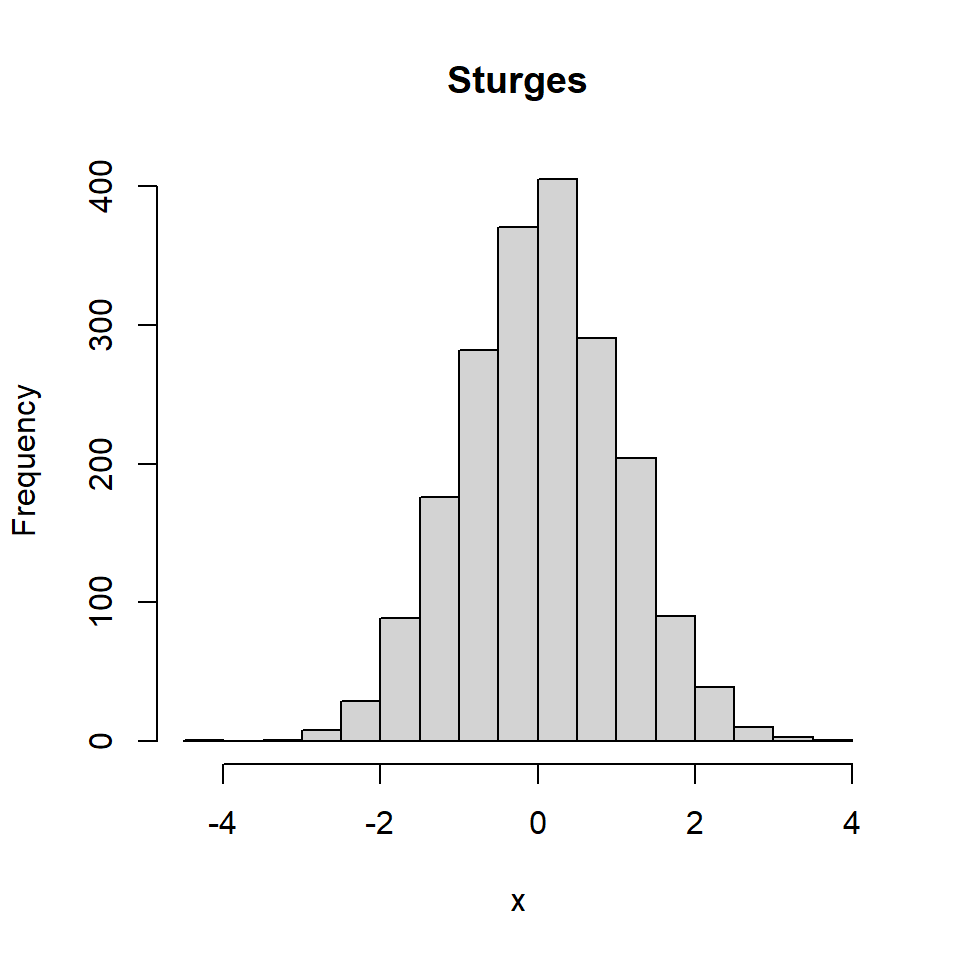Too many bins

If you specify the number of breaks manually make sure the number is not too high.

``````# Sample data
set.seed(2)
x <- rnorm(2000)

# Histogram
hist(x, breaks = 80,
main = "Too many bins")``````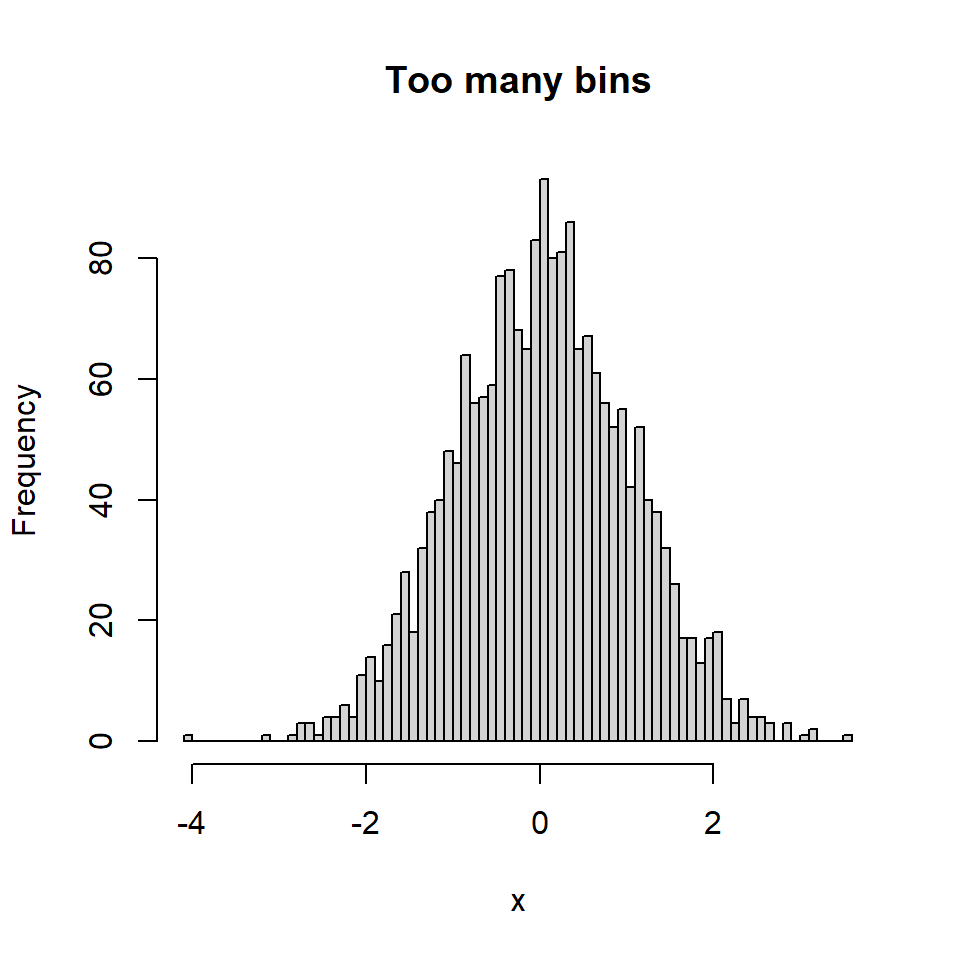Not enough bins

The number of bins can also be too small in some cases.

``````# Sample data
set.seed(2)
x <- rnorm(2000)

# Histogram
hist(x, breaks = 5,
main = "Not enough bins")``````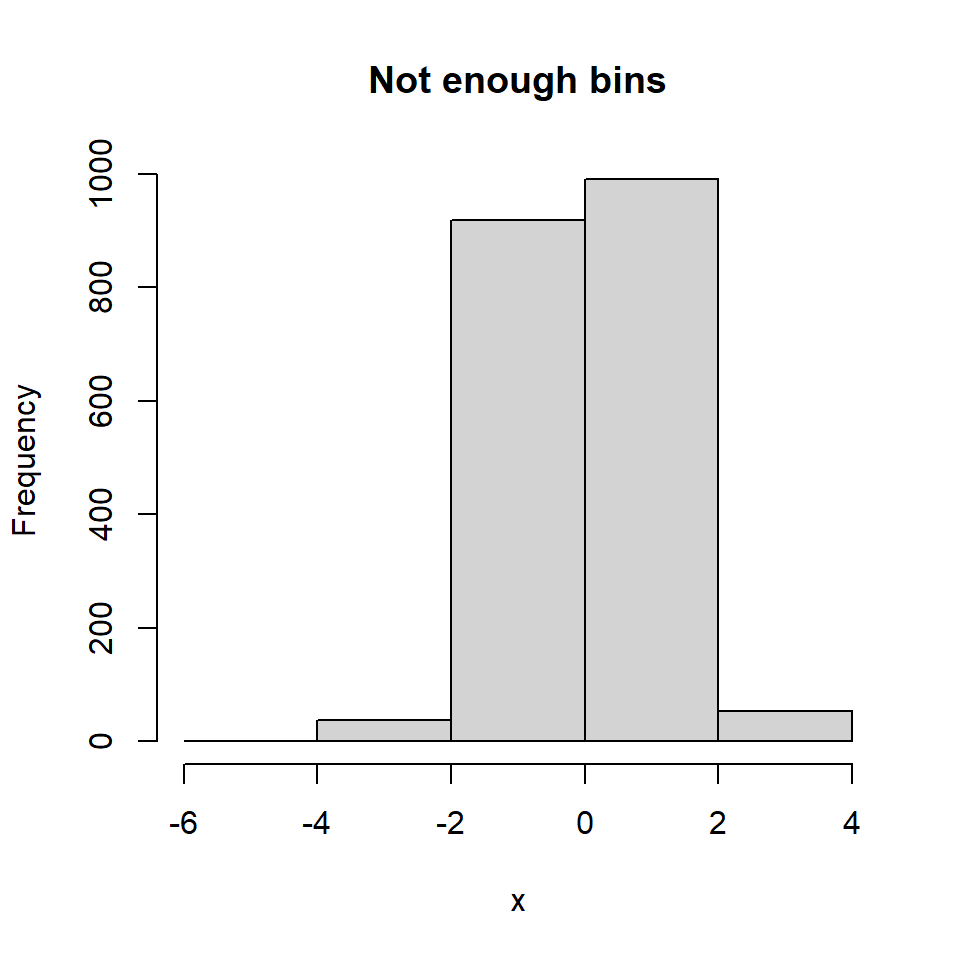Scott method

In addition to the Sturges method the `breaks` argument also supports the Scott method.

``````# Sample data
set.seed(2)
x <- rnorm(2000)

# Histogram
hist(x, breaks = "Scott",
main = "Scott")``````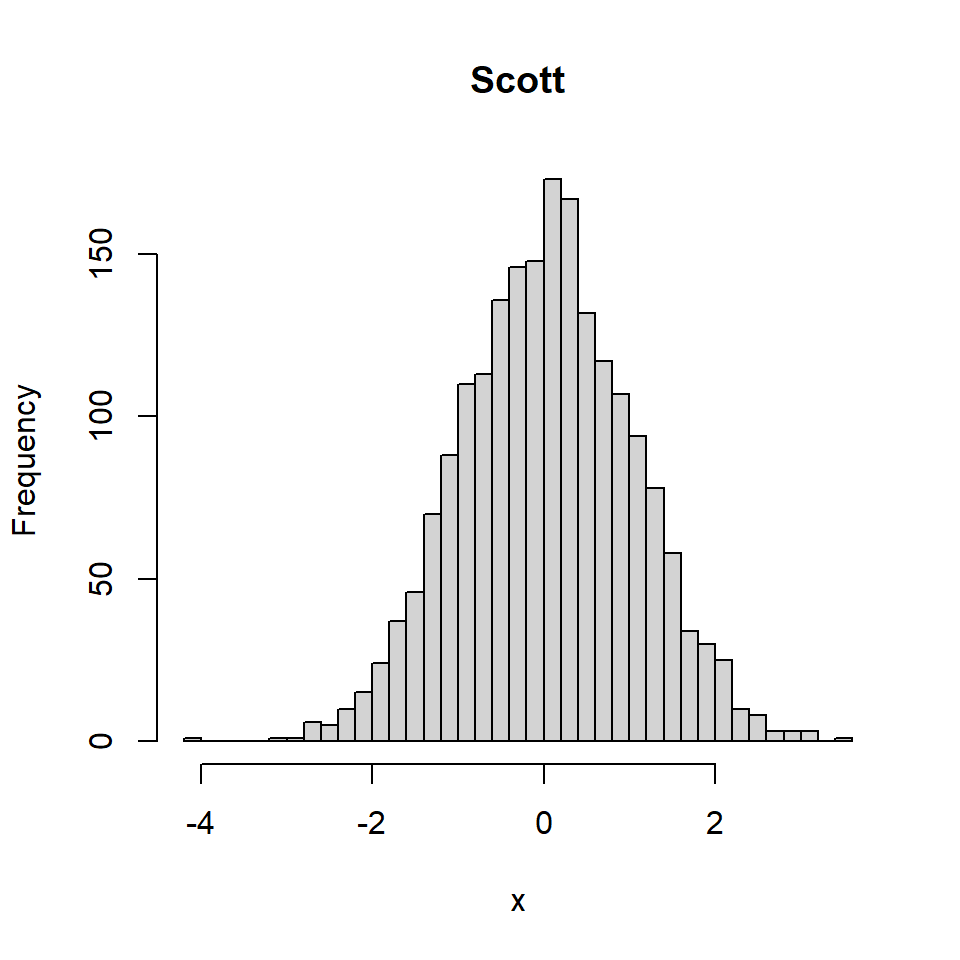Freedman-Diaconis (FD) method

The Freedman-Diaconis algorithm can be selected passing “Freedman-Diaconis” or “FD” to the argument.

``````# Sample data
set.seed(2)
x <- rnorm(2000)

# Histogram
hist(x, breaks = "Freedman-Diaconis",
main = "Freedman-Diaconis")
hist(x, breaks = "FD", # Equivalent
main = "Freedman-Diaconis") ``````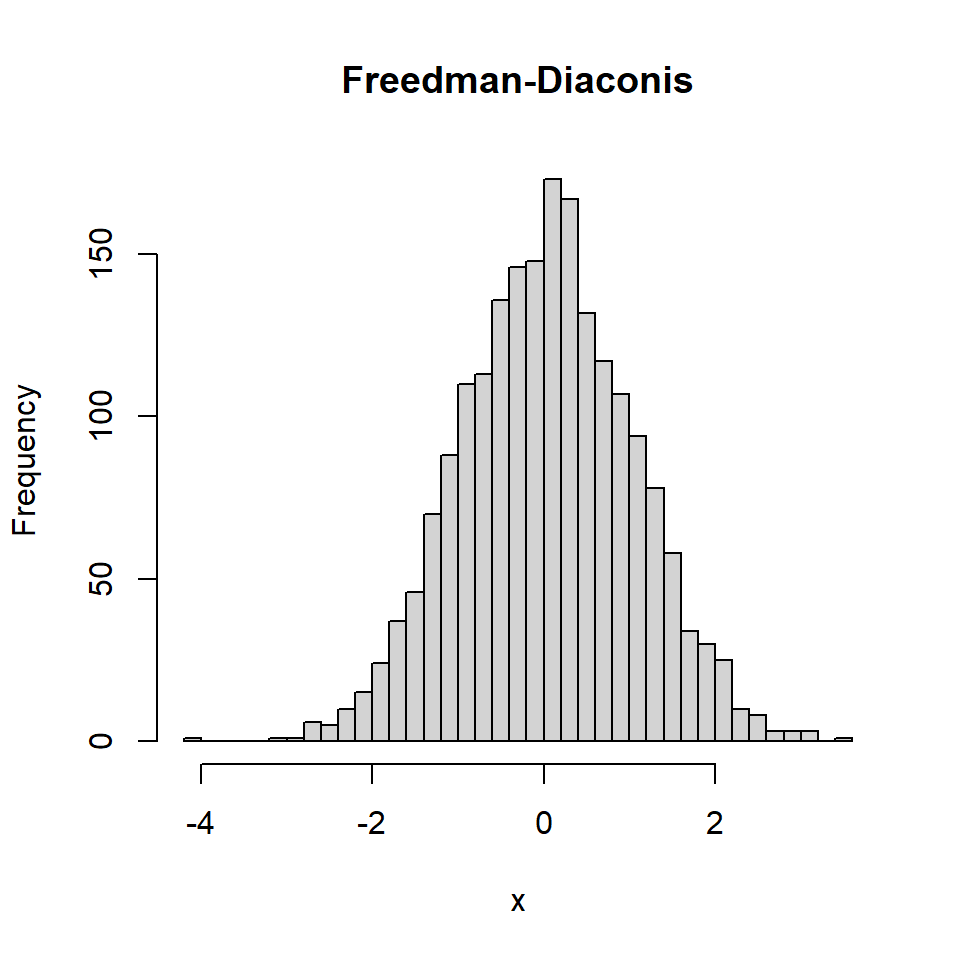You can also pass a vector giving the number of breakpoints or a function to compute the number of bins or breakpoints.

## Plug in selection

An alternative to the Sturges method and selecting the breaks argument by hand is using the plug-in method to calculate the optimal bandwidth (Wand, 1995). This method is implemented in `KernSmooth` and you can use it as follows.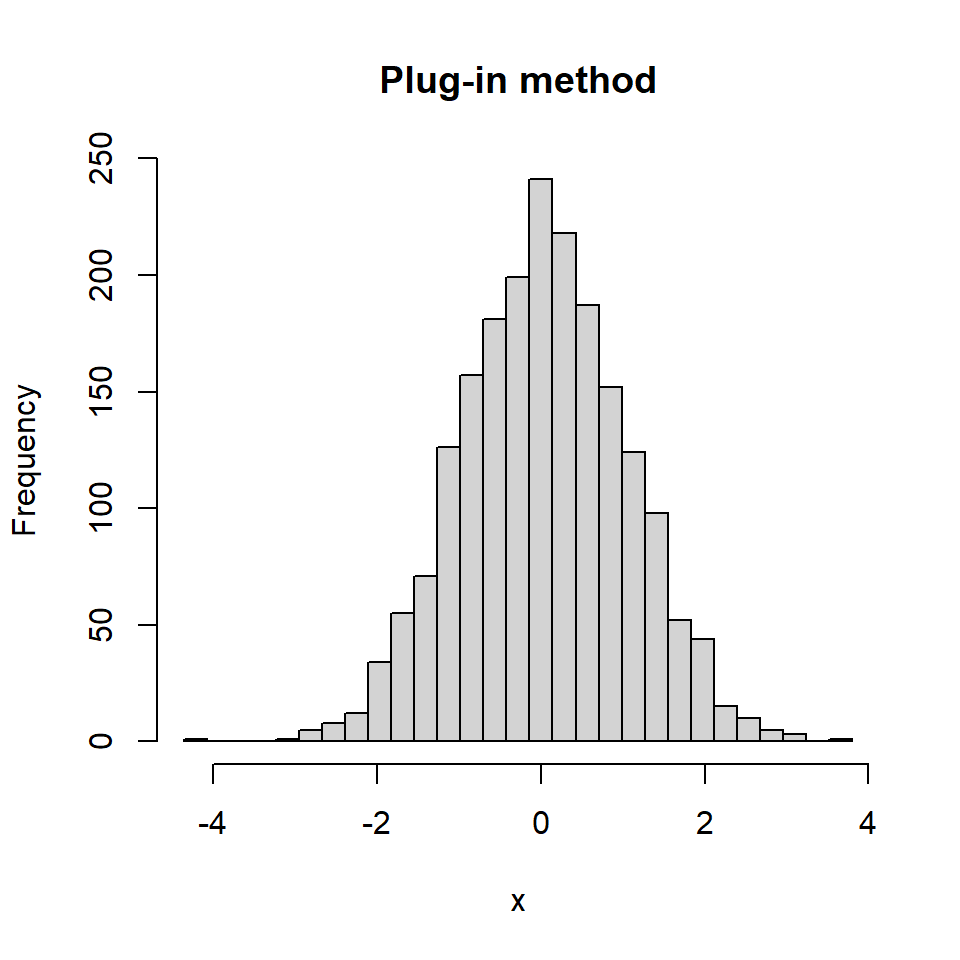``````# Sample data
set.seed(2)
x <- rnorm(2000)

# install.packages("KernSmooth")
library(KernSmooth)

# Optimal bandwidth
bin_width <- dpih(x)

# Number of bins
nbins <- seq(min(x) - bin_width,
max(x) + bin_width,
by = bin_width)

# Histogram
hist(x, breaks = nbins,
main = "Plug-in method")``````